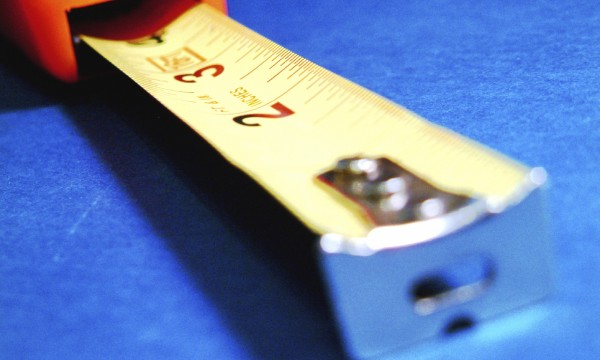# How do I estimate the size of my roof?

October 15, 2014

Whether you’re on top of your house with rectangles and triangles, or estimating from the ground with pitch, here are two ways to calculate the area of your roof.
You need to get a new roof. Yes, the estimates you get from professional roofers will include a calculation of your roof area. But a conscientious homeowner will do his own roof area measurement to ensure that no costly mistakes are made.

To measure the roof, you’ll need to know how to find the areas of basic rectangle and triangle shapes, or perhaps how to calculate roof pitch.
When calculating roof size, you should always take into account wastage during construction. So for a very simple roof, you should add five per cent for waste. This should go up to 10 per cent for a slightly more complicated roof, and 15 per cent for ones even more so (with lots of valleys and dormers, or complicated shapes, for example).
On the roof — get the picture
If you are comfortable getting right up on the roof, and can do so safely, then this is the most accurate way to measure its area. But before you climb up, make a rough diagram of your roof, dividing it into rectangle and triangle areas.
Calculating the areas
Once on the roof, use a tape measure to calculate the length and width of each rectangular area. Then multiply these two numbers together to give you the rectangle’s area. Note these figures on your diagram.

For a triangular area, multiply the width of the base by the triangle’s height and divide this number by two to give you the area. Again, make a note on your handy diagram.
Add up all the area values to give you your roof’s square footage. Roofing materials are determined by the number of “squares” a roof is. To calculate the square of a roof, take the number of square feet you came up with and divide it by 100. A 3,000-square-foot roof would be 30 squares.
From the ground
While measuring from the ground isn’t as accurate as going up on the roof itself, it is safer and does give you a rough idea to compare with roofer estimates. Assuming you have a rectangular shaped house, use a tape measure to measure its length and width from the edges of the eaves. Then multiply these two numbers together.

If the house shape is complex, you will have to calculate each wing or room separately.
What’s the pitch?
But hold on, you’re not finished yet. Unless you have a flat roof, you’ll need to calculate your roof pitch.

Roof pitch is determined by the number of inches it rises vertically for every 12 inches it extends horizontally. We’re afraid you’ll brieflyhave to go onto the roof itself for this. Mark a level at 12 inches, hold it perfectly level and measure from the roof surface to the 12 inch mark. A roof with a 3 inch rise (known as the roof’s slope or its slant) over 12 inches would be marked as having a pitch of 3/12. You would then use a calculator to determine the decimal figure — 1.03.
Take the original number for your ground level square footage and multiply it by your pitch decimal figure to give your “roof square.”

With final number proudly in hand, see how your estimate squares with the roofer’s, and then let the building begin!## How much paint do you need? Some handy tips

The material on this website is provided for entertainment, informational and educational purposes only and should never act as a substitute to the advice of an applicable professional. Use of this website is subject to our terms of use and privacy policy.